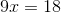# ISEE Upper Level Math : How to find the length of the side of an acute / obtuse isosceles triangle

## Example Questions

### Example Question #1 : Triangles

Two sides of an isosceles triangle have lengths 3 feet and 4 feet. Which of the following could be the length of the third side?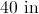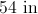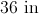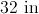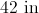Explanation:

An isosceles triangle, by definition, has two sides of equal length. Having the third side measure either 3 feet or 4 feet would make the triangle meet this criterion.

3 feet is equal to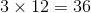inches, and 4 feet is equal to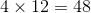inches. We choose 36 inches, since that, but not 48 inches, is a choice.

### Example Question #1 : How To Find The Length Of The Side Of An Acute / Obtuse Isosceles Triangle

The triangles are similar. Solve for.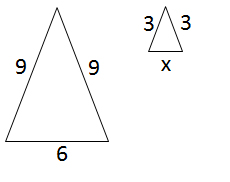Explanation:

Because the triangles are similar, proportions can be used to solve for the length of the side: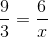Cross-multiply: